# 7 WAYS TO USE EXCEL REPT FUNCTION

When is the last time you used REPT function in Excel?

REPT  is one of excel’s little-known, overlooked and underutilized function, yet very useful.

Generally, REPT  returns a specific text string a specified number of times.

=REPT(“Text”, Number of times)

Here are 7 ways you can start using REPT function.

• ADD LEADING ZEROS
• CREATE INLINE CHARTS
• REPLACE NESTED IF
• CREATE A MIRROR GRAPH
• CREATE-STEM AND LEAF PLOTS
• EXTRACT N WORDS FROM A TEXT STRING
• CREATE A STAR RATING SYSTEM

# ADD LEADING ZEROS

By default, Excel removes any leading zero in a number yet there are instances where these are required.

For example, suppose you want to convert numbers to cost codes? or store phone

Note, the cost codes should start with a zero and must have 10 characters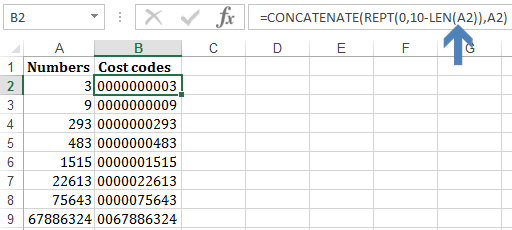`=CONCATENATE(REPT(0,10-LEN(A2)),A2)`

How it works:

►10-LEN(A2)–Calculates the number of times to repeat Zero.

Since we need 10 characters in the cell, then the number of times the leading zeros are repeated should be 10 minus the length of the numbers.

CONCATENATE function just joins the repeated zeros with the number. You can also use an ampersand to join the two

`=REPT(0,10-LEN(A9))&A9`

# CREATE INLINE CHARTS

Inline charts are very important in creating dashboards. With simple REPT function, you can build a dynamic inline chart as shown below=REPT(“|”,B2*10)

How it works

► After inserting the formula on row 3, change font to Playbill, make it bold with size 28

►Reallign the contents in row 3 to 90° .  Go to cell format, alignment, set to 90 degrees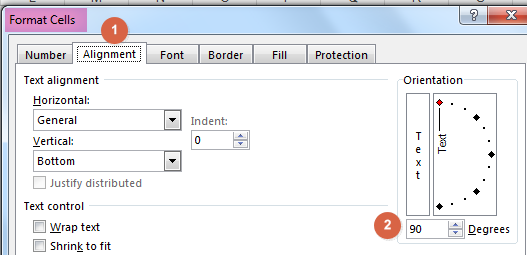►Apply conditional formatting to the charts based on the costing percentages.  Go to conditional formatting, manage rules, new rule, then set your rules.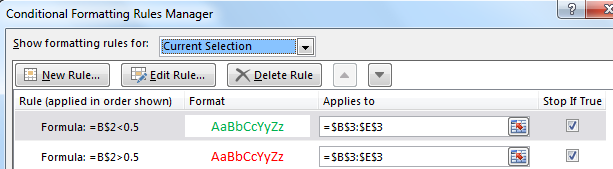# REPLACE NESTED IF

As shown in this article, 7 alternatives to Nested IF, you can speed your spreadsheet calculations by replacing nested IF functions with REPT

For example, suppose you have below production figures and you want to return it the status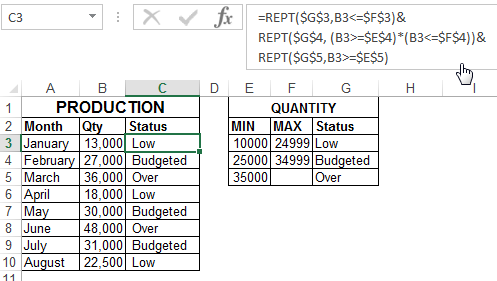```=REPT(\$G\$3,B3<=\$F\$3)&
REPT(\$G\$4, (B3>=\$E\$4)*(B3<=\$F\$4))&
REPT(\$G\$5,B3>=\$E\$5)```

How it works

Excel recognizes internally the value of TRUE as number 1 and value of FALSE as 0.  Also, REPT functions return an empty string if the number to return is zero. These are the fact that REPT utilizes in above function.

Firstly, REPT function evaluates every condition and returns a TRUE or FALSE.

Since ONLY 1  condition will evaluate TRUE, REPT function returns a string of empty strings and one status value.

`="Low"&""&""`

# CREATE A MIRROR GRAPH

Mirror graphs are among the hardest to create in my opinion yet among the best in comparing 2 sets of data.

I had been struggling to find an easy and flexible way until I found REPT function.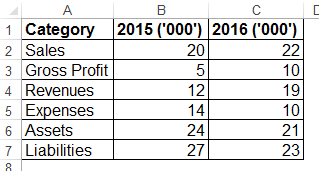For example, how do you transform above data to below mirror chart?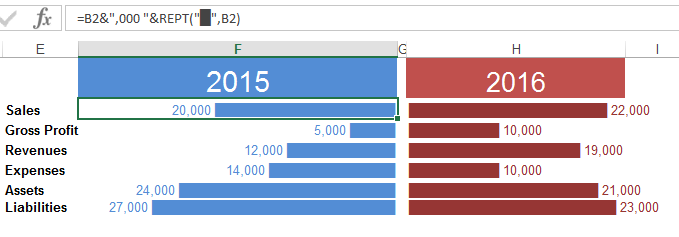Steps:

► Insert REPT function in Column F. On the text to repeat type “Alt+220” this will repeat this character ▄

►On the numbers to repeat, reference the units per category and join them with “,000” text string.

► For the data in column F, ensure you align your content to the right.

►Change the text font appropriately.

# CREATE STEM & LEAF PLOTS

A Stem and Leaf Plot is a special table where each data value is split into a “stem” (the first digit or digits) and a “leaf” (usually the last digit). More like a frequency distribution table.

For example, how do you create a stem & leaf plot for below quantity data?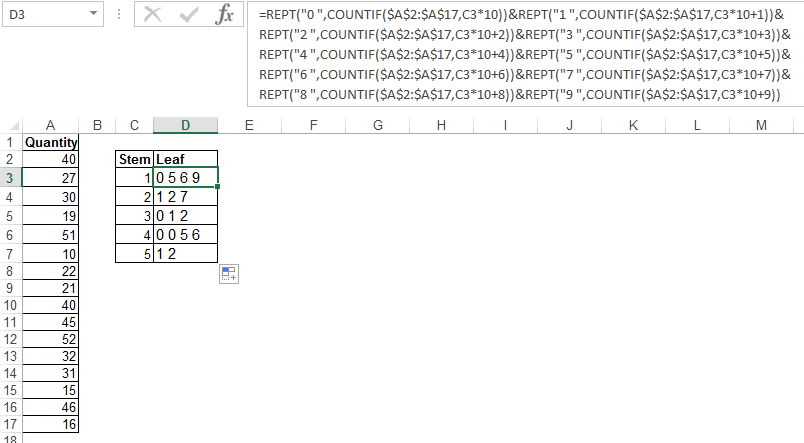```=REPT("0 ",COUNTIF(\$A\$2:\$A\$17,C3*10))&REPT("1 ",COUNTIF(\$A\$2:\$A\$17,C3*10+1))&
REPT("2 ",COUNTIF(\$A\$2:\$A\$17,C3*10+2))&REPT("3 ",COUNTIF(\$A\$2:\$A\$17,C3*10+3))&
REPT("4 ",COUNTIF(\$A\$2:\$A\$17,C3*10+4))&REPT("5 ",COUNTIF(\$A\$2:\$A\$17,C3*10+5))&
REPT("6 ",COUNTIF(\$A\$2:\$A\$17,C3*10+6))&REPT("7 ",COUNTIF(\$A\$2:\$A\$17,C3*10+7))&
REPT("8 ",COUNTIF(\$A\$2:\$A\$17,C3*10+8))&REPT("9 ",COUNTIF(\$A\$2:\$A\$17,C3*10+9))```

How it works:

The formula is scary big but fairly easy to understand once you break it down:

►COUNTIF(\$A\$2:\$A\$17,C3*10)–counts how many tens, the twenties, thirties e.t.c occur in the range.

►REPT(“0 “,COUNTIF(\$A\$2:\$A\$17,C3*10))–returns Zero the number of times these tens, twenties e.t.c occur

►COUNTIF(\$A\$2:\$A\$17,C3*10+1)–to cater for the occurrences ones of these tens, the twenties e.t.c, we add either 1, 2 , 3, 4, 5, 9, 7, 8, 9 in our COUNTIF function

►REPT(“1 “,COUNTIF(\$A\$2:\$A\$17,C3*10+1))–for example, this part of the formula returns 1 the number of times 11 occurs

NB: If you need more explanation Watch this Video

# EXTRACT n WORDS FROM A TEXT STRING

It is easy to extract characters from a string if you know the numbers of characters to extract. MID, RIGHT, and LEFT functions are mostly used in these string manipulation.

MID, RIGHT, and LEFT functions are mostly used in these string manipulation.

But, how do you extract the last 3 words from below sentences? how do you find the 3rd single space, from the right, and extract all words after it?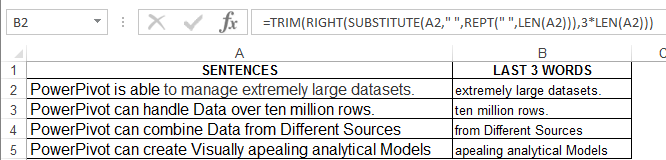`=TRIM(RIGHT(SUBSTITUTE(A2," ",REPT(" ",LEN(A2))),3*LEN(A2)))`

How it Works

Since the last 3 words are of different lengths from sentence to sentence, we need to find a way to use the 3rd last single space as our criteria to extract them.

REPT(” “, LEN(A2))–takes all single spaces in the sentence repeats them a number of times equal to the length of the sentence.

SUBSTITUTE(A2,” “, REPT(” “, LEN(A2)))–finds the single spaces and replaces them with the repeated spaces from REPT function.

Since you now know the relative position of the spaces, you can use RIGHT function and the LEN function to find the last 3 words

RIGHT(SUBSTITUTE(A2,” “,REPT(” “,LEN(A2))),3*LEN(A2))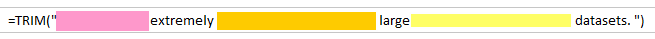Since the words extracted by RIGHT function still has the repeated spaces, use the TRIM function to cut all extra spaces except single spaces between words.

NB: For more explanations, WATCH THIS VIDEO

# CREATE A STAR RATING TEMPLATE`=IF(B3="",REPT(\$E\$2,5),REPT(\$D\$2,B3)&REPT(\$E\$2,5-B3))`

How it works:

Go to Insert ►Symbols ► Select Wingdings under font ►Insert two starts (☆★) on separate cells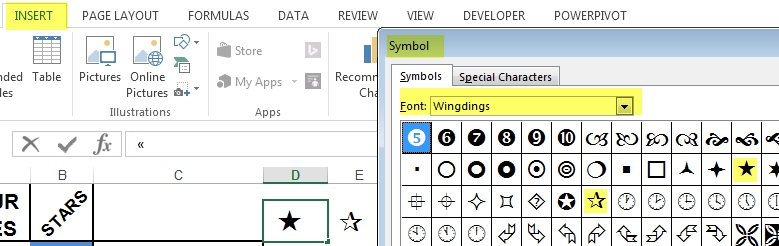IF(B3=””,REPT(\$E\$2,5)–in case the ratings are blank,repeats the blank star 5 times

REPT(\$D\$2, B3)&REPT(\$E\$2,5-B3)–in the case of a rating, repeat the full star the number of rating score and the blank star 5 minus the rating score.

Edit: 2nd July:

After posting the article on LinkedinAbhijeet R. Joshi suggested a tweak simplifying the star rating.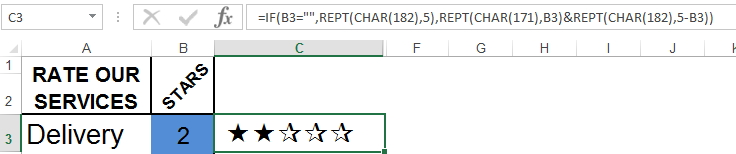Instead of inserting the symbols first, referencing them and repeating them, you can just hard-code them in the function.

`=IF(B3="",REPT(CHAR(182),5),REPT(CHAR(171),B3)&REPT(CHAR(182),5-B3))`

Ensure you change the font in column C to Wingdings.

NB: Check out an alternative of creating these star rating

DOWNLOAD WORKSHEET

## Related Links

7 OVERLOOKED USES OF EXCEL MOD FUNCTION

EXCEL’S WEEKDAY FUNCTION IN DEPTH

SUMPRODUCT–EXCEL’S MOST POWERFUL FUNCTION

## Recommended BookCheck it Out

## Inspiration Sites for the Article:

Read more on Stem & Leaf Plots

Read more on Inner charts

Read more on uses of REPT Functions